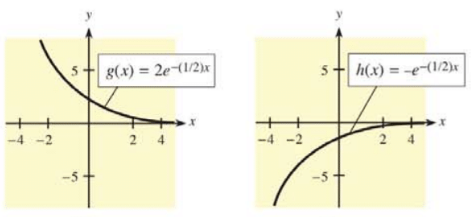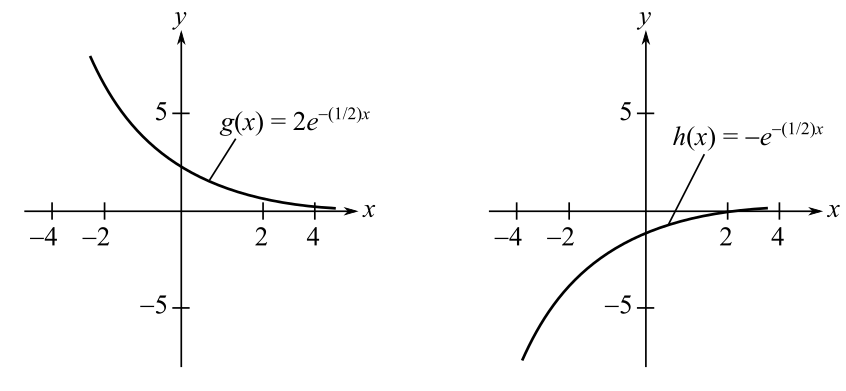Chapter 12.3, Problem 33E### Mathematical Applications for the ...

11th Edition
Ronald J. Harshbarger + 1 other
ISBN: 9781305108042

#### Solutions

Chapter
Section### Mathematical Applications for the ...

11th Edition
Ronald J. Harshbarger + 1 other
ISBN: 9781305108042
Textbook Problem

# In Problems 33 and 34, graphs of two functions labeled g(x) and h(x) are given. Decide which is the graph of f(x) and which is one member of the family ∫ f ( x ) d x . Check your conclusions by evaluating the integral.To determine

The explanation which one is the graph of f(x) and which one is the member of the family f(x)dx from the provided graphs of two functions g(x) and h(x):Explanation

Given information:

The provided graphs are:

The provided functions are: g(x)=2e(12)x and h(x)=e(12)x

Explanation:

It is provided that h(x)=e(12)x.

Consider that h(x)=f(x)=e(12)x

Integrate the function f(x)=e(12)x and further solve as:

f(x)dx=(e(12)x

### Still sussing out bartleby?

Check out a sample textbook solution.

See a sample solution

#### The Solution to Your Study Problems

Bartleby provides explanations to thousands of textbook problems written by our experts, many with advanced degrees!

Get Started

#### solve the equation by using the quadratic formula. 135. 4x2 + 5x 6 = 0

Applied Calculus for the Managerial, Life, and Social Sciences: A Brief Approach

#### In Exercises 516, evaluate the given quantity. log218

Finite Mathematics and Applied Calculus (MindTap Course List)

#### Which graph is best described by a linear model?

Study Guide for Stewart's Single Variable Calculus: Early Transcendentals, 8th

#### Find for y defined implicity by .

Study Guide for Stewart's Multivariable Calculus, 8th